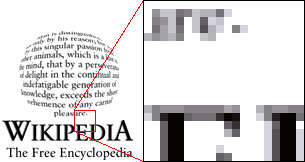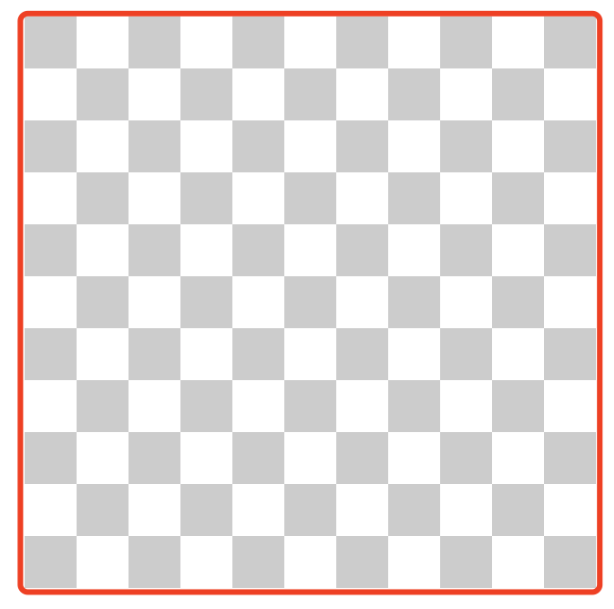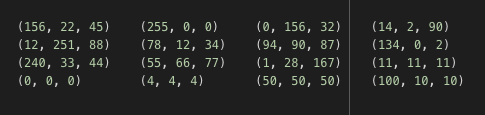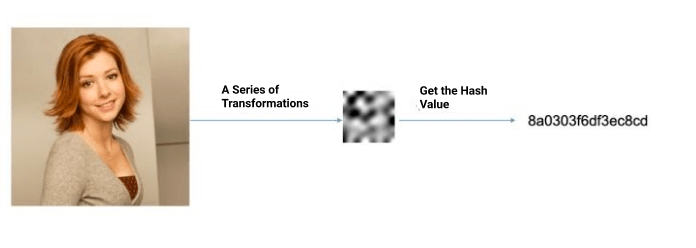August 04, 2020by Rife Wang

# The Journey to Optimizing Billion-scale Image Search (1/2)

• scenarios
A case study with UPYUN. Learn about how Milvus stands out from traditional database solutions and helps to build an image similarity search system.

Yupoo Picture Manager serves tens of millions of users and manages tens of billions of pictures. As its user gallery is growing larger, Yupoo has an urgent business need for a solution that can quickly locate the image. In other words, when a user inputs an image, the system should find its original image and similar images in the gallery. The development of the search by image service provides an effective approach to this problem.

The search by image service has undergone two evolutions:

1. Began the first technical investigation in early 2019 and launched the first-generation system in March and April 2019;
2. Began the investigation of the upgrade plan in early 2020 and started the overall upgrade to the second-generation system in April 2020.

This article describes the technology selection and basic principles behind the two generations of search by image system based on my own experience on this project.

## Overview

### What is an image?

We must know what is an image before dealing with images.

The answer is that an image is a collection of pixels.

For example, the part in the red box on this image is virtually a series of pixels.Suppose the part in the red box is an image, then each independent small square in the image is a pixel, the basic information unit. Then, the size of the image is 11 x 11 px.### Mathematical representation of images

Each image can be represented by a matrix. Each pixel in the image corresponds to an element in the matrix.

### Binary images

The pixels of a binary image is either black or white, so each pixel can be represented by 0 or 1. For example, the matrix representation of a 4 * 4 binary image is:

``````0 1 0 1
1 0 0 0
1 1 1 0
0 0 1 0
``````

### RGB images

The three primary colors (red, green, and blue) can be mixed to produce any color. For RGB images, each pixel has the basic information of three RGB channels. Similarly, if each channel uses an 8-bit number (in 256 levels) to represent its gray scale, then the mathematical representation of a pixel is:

``````([0 .. 255], [0 .. 255], [0 .. 255])
``````

Taking a 4 * 4 RGB image as an example:The essence of image processing is to process these pixel matrices.

## The technical problem of search by image

If you are looking for the original image, that is, an image with exactly the same pixels, then you can directly compare their MD5 values. However, images uploaded to the Internet are often compressed or watermarked. Even a small change in an image can create a different MD5 result. As long as there is inconsistency in pixels, it is impossible to find the original image.

For a search-by-image system, we want to search for images with similar content. Then, we need to solve two basic problems:

• Represent or abstract an image as a data format that can be processed by a computer.
• The data must be comparable for calculation.

More specifically, we need the following features:

• Image feature extraction.
• Feature calculation (similarity calculation).

## The first-generation search-by-image system

### Feature extraction — image abstraction

The first-generation search-by-image system uses Perceptual hash or pHash algorithm for feature extraction. What are the basics of this algorithm?As shown in the figure above, the pHash algorithm performs a series of transformations on the image to get the hash value. During the transformation process, the algorithm continuously abstract images, thereby pushing the results of similar images closer to each other.

### Feature calculation — similarity calculation

How to calculate the similarity between the pHash values of two images? The answer is to use the Hamming distance. The smaller the Hamming distance, the more similar the images’ content.

What is Hamming distance? It is the number of different bits.

For example,

``````Value 1： 0 1 0 1 0
Value 2： 0 0 0 1 1
``````

There are two different bits in the above two values, so the Hamming distance between them is 2.

Now we know the principle of similarity calculation. The next question is, how to calculate the Hamming distances of 100-million-scale data from 100-million-scale pictures? In short, how to search for similar images?

In the early stage of the project, I did not find a satisfactory tool (or a computing engine) that can quickly calculate the Hamming distance. So I changed my plan.

My idea is that if the Hamming distance of two pHash values is small, then I can cut the pHash values and the corresponding small parts are likely to be equal.

For example:

``````Value 1： 8 a 0 3 0 3 f 6
Value 2： 8 a 0 3 0 3 d 8
``````

We divide the above two values into eight segments and the values of six segments are exactly the same. It can be inferred that their Hamming distance is close and thus these two images are similar.

After the transformation, you can find that the problem of calculating Hamming distance has become a problem of matching equivalence. If I divide each pHash value into eight segments, as long as there are more than five segments that have exactly the same values, then the two pHash values are similar.

Thus it is very simple to solve equivalence matching. We can use the classical filtering of a traditional database system.

Of course, I use the multi-term matching and specify the degree of matching using minimum_should_match in ElasticSearch (this article does not introduce the principle of ES, you can learn it by yourself).

Why do we choose ElasticSearch? First, it provides the above-mentioned search function. Second, the image manager project in itself is using ES to provide a full-text search function and it is very economical to use the existing resources.

## Summary of the first-generation system

The first-generation search-by-image system chooses the pHash + ElasticSearch solution, which has the following features:

• The pHash algorithm is simple to use and can resist a certain degree of compression, watermark, and noise.
• ElasticSearch uses the existing resources of the project without adding additional costs to the search.
• But the limitation of this system is obvious: The pHash algorithm is an abstract representation of the entire image. Once we destroy the integrity of the image, such as adding a black border to the original image, it is almost impossible to judge the similarity between the original and the others.

To break through such limitations, the second-generation image search system with a completely different underlying technology emerged.